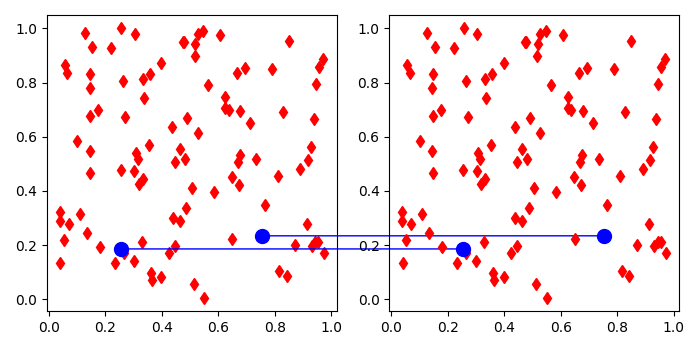# Drawing lines between two plots in Matplotlib

To draw lines between two plots in matplotlib, we can take the following steps −

• Create a new figure or activate an existing figure.

• Add two axes (ax1 and ax2) to the figure as part of a subplot arrangement.

• Create random data x and y using numpy.

• Plot x and y data points on both the axes (ax1 and ax2) with color=red and marker=diamond.

• Initialize two variables, i and j to get the diffirent data points on the subplot.

• Make xy and mn tuple for positions to add a patch on the subplots.

• Add a patch that connects two points (possibly in different axes), con1 and con2.

• Add artists for con1 and con2.

• Plot lines on ax1 and ax2 with data points (x[i], y[i]) and (x[j], y[j]), to interlink the patches.

• To display the figure, use show() method.

## Example

import matplotlib.pyplot as plt
from matplotlib.patches import ConnectionPatch
import numpy as np
plt.rcParams["figure.figsize"] = [7.00, 3.50]
plt.rcParams["figure.autolayout"] = True
fig = plt.figure()
x, y = np.random.rand(100), np.random.rand(100)
ax1.plot(x, y, 'd', c='r')
ax2.plot(x, y, 'd', c='r')
i = 10
xy = (x[i], y[i])
con1 = ConnectionPatch(xyA=xy, xyB=xy, coordsA="data", coordsB="data", axesA=ax2, axesB=ax1, color="blue")
j = 12
mn = (x[j], y[j])
con2 = ConnectionPatch(xyA=mn, xyB=mn, coordsA="data", coordsB="data", axesA=ax2, axesB=ax1, color="blue")
plt.show()